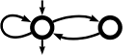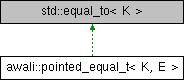Awali Another Weighted Automata library
awali::pointed_equal_t< K, E > Class Template Reference

This class takes a type `E` that computes equality for type `K` and allows to compute equality for type `K const*` by calling deferencing both values and calling `E` on them. More...

`#include <linked_map.hxx>`

Inheritance diagram for awali::pointed_equal_t< K, E >:## Public Member Functions

bool operator() (K *key1, K *key2) const noexcept

## Detailed Description

### template<typename K, typename E = std::equal_to<K>> class awali::pointed_equal_t< K, E >

This class takes a type `E` that computes equality for type `K` and allows to compute equality for type `K const*` by calling deferencing both values and calling `E` on them.

A `nullptr` is considered equal to itself and different to other pointers.

## ◆ operator()()

template<typename K , typename E = std::equal_to<K>>
 bool awali::pointed_equal_t< K, E >::operator() ( K * key1, K * key2 ) const
noexcept

The documentation for this class was generated from the following file: